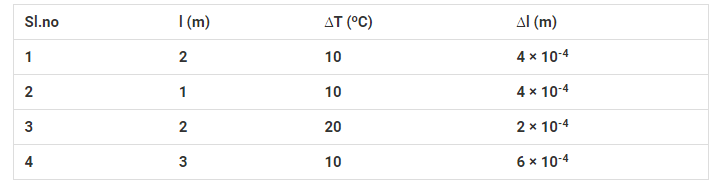# A student records the initial length l,`
Question:

A student records the initial length l, change in temperature ∆T and change in length ∆l of a rod as follows:If the first observation is correct, what can you say about observations 2, 3, and 4.

Solution:

It is given that the first observation is correct which means that the coefficient of linear expansion is

$\alpha=\frac{\Delta l}{l \times \Delta T}=\frac{4 \times 10^{-4}}{2 \times 10}=2 \times 10^{-50} \mathrm{C}^{-1}$

For second observation,

$\Delta l=\alpha l \Delta T=2 \times 10^{-4} m \neq 4 \times 10^{-4} m$

Which is incorrect

For third observation,

$\Delta l=\alpha l \Delta T=8 \times 10^{-4} \mathrm{~m} \neq 2 \times 10^{-4} \mathrm{~m}$

Which is incorrect

For fourth observation,

$\Delta l=\alpha l \Delta T=6 \times 10^{-4} \mathrm{~m}=6 \times 10^{-4} \mathrm{~m}$

Which is correct